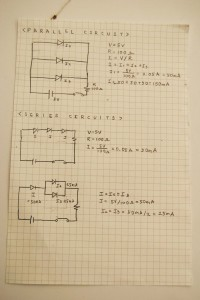# Assignment5

I uploaded 3 videos in vimeo

Parallel Circuit

Series Circuit

Parallel+Series circuit

Schematics + CalculationsIf I calculate with LED resistor,

every LED has resistor and my LED resistor is 3V so

1. Current of first parallel circuit will be

5v-3v=2v

I=V/R

I=2v/100ohm

20mA+20mA+20mA=60mA

2. current of second series circuit will be 20mA.

3. current of third parallel and series circuit will be

I=20mA

I2=10mA

I3=10mA

I am taking Physical Computing so it was not really new and it is fun. From Pcom class everyone is good at arduino and the progress of class is fast

so I had hardtime to catch up. I think this cclab with arduino will really helpful to understand circuit.

Also I haven’t calculated ohm’s law before so it makes me to understand more about resistor voltage and current.